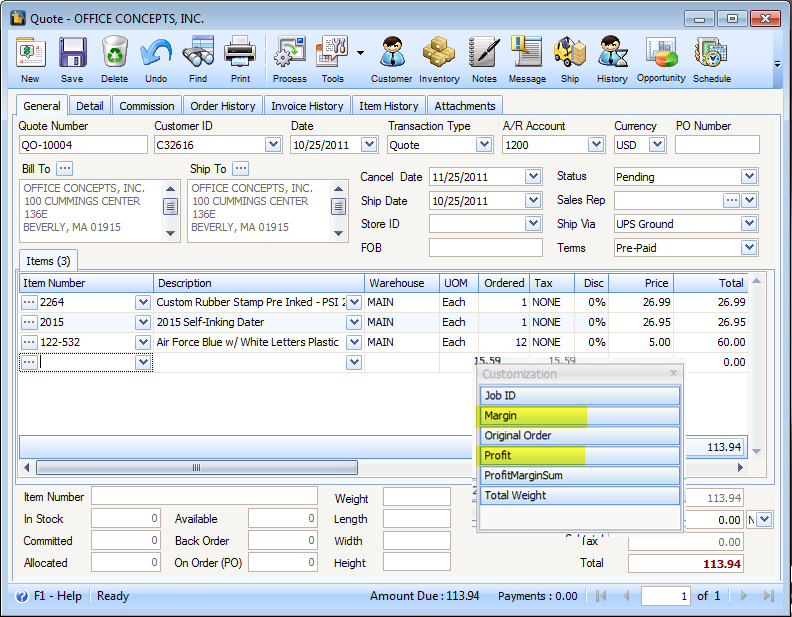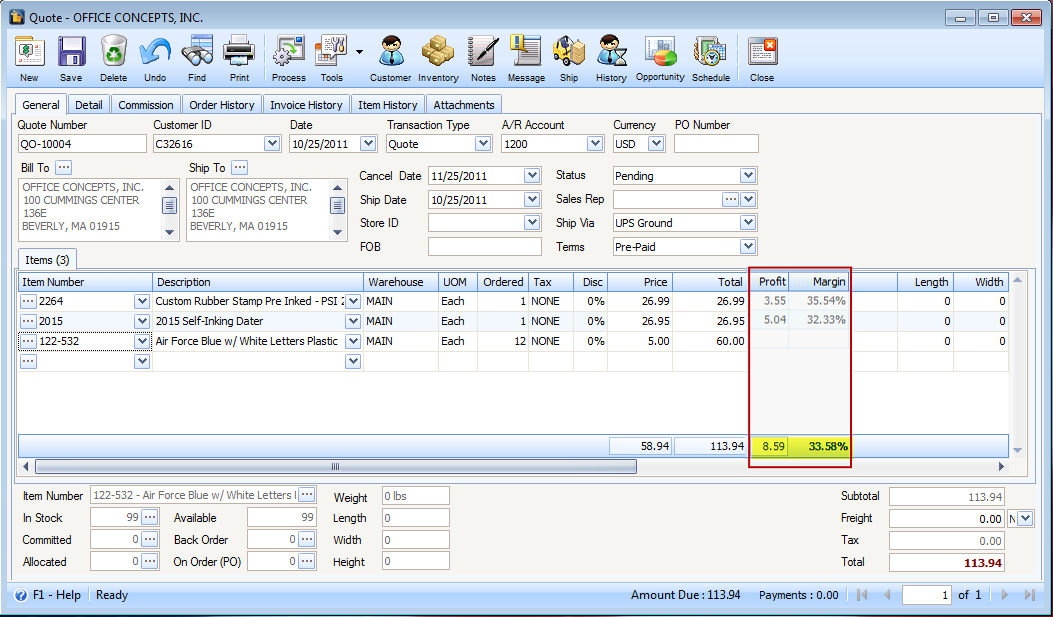﻿ Total Profit and Total Margin fields

# Total Profit and Total Margin fields

On Sales Quote, Order, Invoice, RMA and Credit Memo, added a total field for the Profit and Margin columns.

By default, Profit and Margin columns are not shown in the above mentioned forms.  You will have to add those first.  To do that, on the column header, right-click and select Column Chooser.When Customization mini-form opens, drag the Profit and Margin fields onto the grid area.Profit’s computation:

Price – Cost = Profit

For 2264:

26.99 – 23.44 = 3.55

For 2015:

26.95 – 21.91 = 5.04

Margin’s Computation:

Profit / Price =Margin rate

For 2264:

3.55 / 26.99 = 35.54%

For 2115:

5.04 / 26.95 = 32.33%

Total Profit’s computation:

Sum of profit per line item selected

3.55 + 5.04 = 8.59

Total Margin’s computation:

Total Profit / Total Price = Total Margin

8.59 / 25.58 = 33.58%